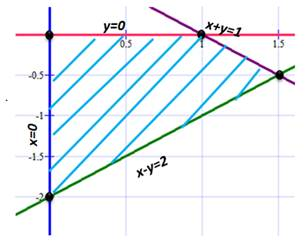### Sample Problem

Find the maximum and minimum values of z=2x+3y subject to the following constraints

x≥0

y≤0

x-y≤2

x+y≤1

maximum value = when x= and y=

minimum value = when x= and y=

#### Solution

First, sketch the region corresponding to the system of constraints. x≥0 is the area to the right of x=0 (the y-axis). y≤0 is the area under y=0 (the x-axis). x-y≤2 is the area above the line y=x-2. x+y≤1 is the area below the line y=1-x. The constraints from the region highlighted in blue stripes.Next, find the vertices of the region

At (0,0): z=2(0)+3(0)=0

At (1, 0): z=2(1)+3(0)=2

At (3/2, -1/2): z=2(3/2)+3(-1/2)=3/2

At (0, -2): z=2(0)+3(-2)=-6

The maximum value of z is 2, and this occurs when x=1 and y=0.

The minimum value of z is -6, and this occurs when x=0 and y=-2.